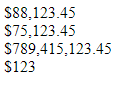GeeksforGeeks App
Open AppBrowser
Continue

# How to format a number as currency using CSS ?

Given a number and the task is to format the number of an HTML element as currency using CSS and a little bit of JavaScript. It is not possible to complete the task with pure CSS. A bit of JavaScript is needed for the parsing of the number to add the commas.

Approach: In the code, the CSS class currSign adds the currency sign (like ‘\$’) before the number. The JavaScript function toLocaleString() returns a string with a language-sensitive representation of the number.

Example: In this example, we are using the above-explained approach.

## html

 `<``style``>``    ``.currSign:before {``        ``content: '\$';``    ``}``` ```<``div` `class``=``"myDIV"``>88123.45``<``div` `class``=``"myDIV"``>75123.45``<``div` `class``=``"myDIV"``>789415123.45``<``div` `class``=``"myDIV"``>123` ```<``script``>``    ``let x = document.querySelectorAll(".myDIV");``    ``for (let i = 0, len = x.length; i < ``len``; i++) {``        ``let ``num` `= ``Number``(x[i].innerHTML)``            ``.toLocaleString('en');``        ``x[i]``.innerHTML` `= ``num``;``        ``x[i].classList.add("currSign");``    ``}```

Output:My Personal Notes arrow_drop_up
Related Tutorials